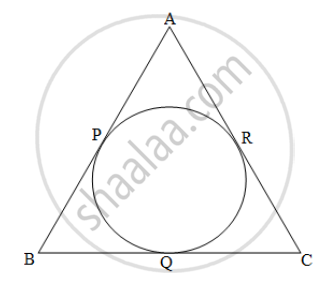# In figure, ΔABC is an isosceles triangle with perimeter 44 cm. The base BC is of length 12 cm. Side AB and side AC are congruent. A circle touches the three sides as shown in the figure below. Find the length of the tangent segment from A to the circle. - Geometry

In figure, ΔABC is an isosceles triangle with perimeter 44 cm. The base BC is of length 12 cm. Side AB and side AC are congruent. A circle touches the three sides as shown in the figure below. Find the length of the tangent segment from A to the circle.#### Solution

Given:- In an isosceles triangle ΔABC side AB ≅ side AC,

Perimeter = 44 cm and base BC = 12 cm.

Perimeter of ΔABC = 44 cm

AB + BC + AC = 44

AB + BC + AB = 44                  .............(side AB ≅ side AC)

2AB + 12 = 44                        ..............(side BC = 12)

2AB = 44 - 12 = 32

AB = 32/2 = 16

AB = AC = 16

Concept: Circumference of a Circle
Is there an error in this question or solution?
2013-2014 (March)

Share